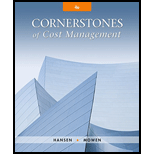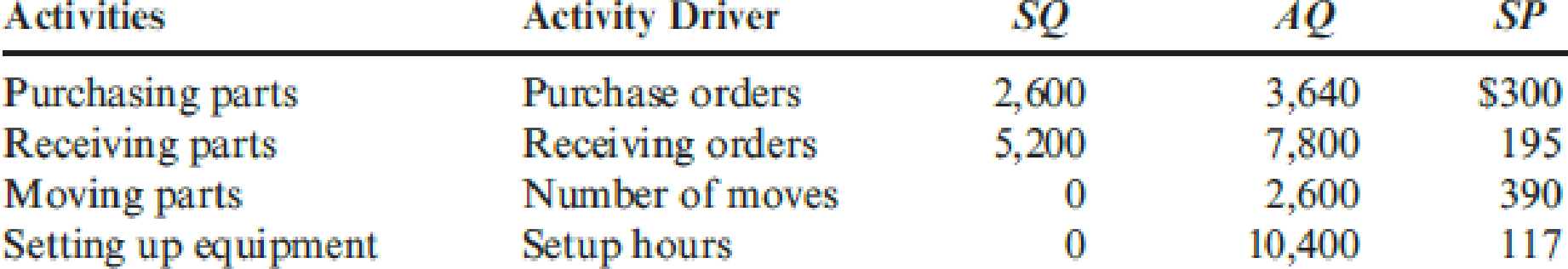# Sanford, Inc., has developed value-added standards for four activities: purchasing parts, receiving parts, moving parts, and setting up equipment. The activities, the activity drivers, the standard and actual quantities, and the price standards for 20x1 are as follows: The actual prices paid per unit of each activity driver were equal to the standard prices. Required: 1. Prepare a cost report that lists the value-added, non-value-added, and actual costs for each activity. 2. Which activities are non-value-added? Explain why. Also, explain why value-added activities can have non-value-added costs.### Cornerstones of Cost Management (C...

4th Edition
Don R. Hansen + 1 other
Publisher: Cengage Learning
ISBN: 9781305970663

#### Solutions

Chapter
Section### Cornerstones of Cost Management (C...

4th Edition
Don R. Hansen + 1 other
Publisher: Cengage Learning
ISBN: 9781305970663
Chapter 12, Problem 14E
Textbook Problem
222 views

## Sanford, Inc., has developed value-added standards for four activities: purchasing parts, receiving parts, moving parts, and setting up equipment. The activities, the activity drivers, the standard and actual quantities, and the price standards for 20x1 are as follows:The actual prices paid per unit of each activity driver were equal to the standard prices.Required: 1. Prepare a cost report that lists the value-added, non-value-added, and actual costs for each activity. 2. Which activities are non-value-added? Explain why. Also, explain why value-added activities can have non-value-added costs.

1.

To determine

Prepare the cost report that lists all the activities.

### Explanation of Solution

Value added activities: Value added activities are the necessary activity remains in the business. Value added activity contribute the customer value and archive the organization need.

Non-value-added activities: Non-value-added activities are the activity of either unnecessary or necessary activities that are inefficient and improvable. For example: moving of goods.

Prepare the cost report.

 S company Value-added and Non-value added cost report For the year December 31, 20x1 Particulars Value-added Non-value added Actual Purchasing the parts $780,000 (a)$312,000 (b) $1,092,000 (c) Receiving the products$1,014,000 (d) $507,000 (e)$1,521,000 (f) Moving parts 0 $1,014,000 (g)$1,014,000 (h) Setting up equipment 0 $1,216,800 (i)$1,216,800 (j) Total $1,794,000$3,049,800 $4,843,800 Table (1) Working notes: 1. a) Calculate the value-added cost for purchasing the parts. Purchasing the parts=SQ×SP=2,600×$300=$780,000 1. b) Calculate the non-value added cost for purchasing the parts. Purchasing the parts=AQSQ×SP=3,6402,600×$300=$312,000 1. c) Calculate the actual cost for purchasing the parts. Purchasing the parts=AQ×SP=3,640×$300=$1,092,000 1. d) Calculate the value-added cost for receiving the products. Receiving the products=SQ×SP=5,200×$195=\$1,014,000

1. e) Calculate the non-value added cost for receiving the products

2.

To determine

List the activities that are non-value added and provide the reason for listed as non-value added. Explain the reason that value-added activities also have non-value added cost.

### Still sussing out bartleby?

Check out a sample textbook solution.

See a sample solution

#### The Solution to Your Study Problems

Bartleby provides explanations to thousands of textbook problems written by our experts, many with advanced degrees!

Get Started

Find more solutions based on key concepts
EFFECTIVE INTEREST RATE You borrow 85,000; the annual loan payments are 8,273.59 for 30 years. What interest ra...

Fundamentals of Financial Management, Concise Edition (with Thomson ONE - Business School Edition, 1 term (6 months) Printed Access Card) (MindTap Course List)

Explain the real balance effect.

Economics (MindTap Course List)

What are the positive and negative effects of specialization?

Foundations of Business (MindTap Course List)

Differentiate between dealer markets and stock markets that have a physical location.

Fundamentals of Financial Management, Concise Edition (MindTap Course List)